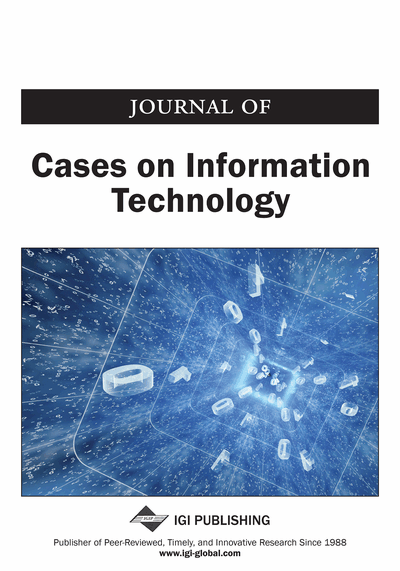# Secure Computation on Cloud Storage: A Homomorphic Approach

Daya Sagar Gupta (Department of Computer Science and Engineering, Indian School of Mines, Dhanbad, India) and G. P. Biswas (Department of Computer Science and Engineering, Indian School of Mines, Dhanbad, India)
DOI: 10.4018/JCIT.2015070103

## Abstract

This paper describes a way by which computation on cloud storage is securely possible. A user stores their secret (encrypted) files on cloud storage and later on, retrieves an addition of their original files, however, the cloud system cannot decrypt the stored encrypted files by own. In this paper, the authors use the homomorphic property to securely compute the addition of the files. The implementation of their proposed protocol is based on the computation on the basic properties of elliptic curves and bilinear mapping. The security of proposed encryption technique depends on the hardness of elliptic curve operations.
Article Preview
Top

## 1. Introduction

The Public key encryption (PKE) plays an important role to encrypt a secret data. This paper uses a PKE technique to ensure the security of the designed protocol. The proposed protocol is based on the homomorphic encryption. The idea of homomorphic encryption is firstly proposed by Rivest, Adleman & Dertouzous (1978) as a notion of privacy homomorphism. A public key encryption technique which includes the homomorphic property: E (m1 oM m2) = E (m1) oC E (m2) is termed as homomorphic encryption. In general, a PKE has three algorithms: keyGen which generates a pair of key (public key and private key), encrypt which encrypts the message using the public key and decrypt which decrypts the message using the private key. Homomorphic encryption also includes these three conventional algorithms with the inclusion of an efficient algorithm evaluate which takes cipher texts c1, c2, ..., cn and public key as inputs and produces a valid encryption of some function f on messages m1, m2, ..., mn i.e.

E (fM (m1, m2..... mn)) <-------------- fC (c1, c2...... cn).

The proposed scheme is based on the elliptic curve cryptography. The elliptic curves play a very important role in the field of cryptography. The security of elliptic curve cryptography is much better than that of the RSA cryptosystem. Our scheme deals with the properties of an elliptic curve. The proposed protocol is based on the bilinear property designed for elliptic curves. The elliptic curve cryptography (ECC) depends on the difficulty provided by elliptic curve operations like addition operation of the points on elliptic curves. Elliptic curve cryptography is nothing but a kind of PKE with a pair of keys i.e. secret and public keys. On the bilinear map, the Computing Diffie-Hellman Problem (CDHP) is difficult, but the Decision Diffie-Hellman Problem (DDHP) is easy. Miller (1985) and Kblitz (1987) independently proposed the security of elliptic curve cryptosystem algorithm which is depending on the discrete logarithm problem of elliptic curves.

In this paper, we are going to present a cryptographic technique which is based on the difficulty of elliptic curve Diffie-Hellman problem and Bilinear Diffie-Hellman problem. The main work is done to secure the cloud storage data. To do so, we use the homomorphic encryption technique in our proposed work. To implement the proposed protocol, we use four algorithms: keyGen, encrypt, decrypt and evaluate. The keyGen is used to generate the public parameters and a secret key. The public parameters are publicly known to others, whereas the secret key is secret to the authenticated user. Encrypt algorithm encrypts the secret message which is then stored on a remote server called a cloud. This encrypted message is not understandable to the remote server, i.e. the message is securely stored in the cloud so that the original message is not visible to the remote server. Decrypt algorithm decrypts the encrypted message to get original message. This decrypt algorithm is only used by the authenticated user so that the only true user can get the original message. The evaluate algorithm is used for a special purpose. This algorithm is used to show the homomorphic property of the proposed protocol. In this paper, user requests to the remote server for the addition of stored messages stored on cloud storage. The cloud system calculates the addition of messages stored and fulfills the request of the client to return the addition calculated. After getting the calculated addition of message from cloud system, the user decrypts the message and finds the addition of the original messages.

## Complete Article List

Search this Journal:
Reset
Open Access Articles
Volume 22: 4 Issues (2020): 2 Released, 2 Forthcoming
Volume 21: 4 Issues (2019)
Volume 20: 4 Issues (2018)
Volume 19: 4 Issues (2017)
Volume 18: 4 Issues (2016)
Volume 17: 4 Issues (2015)
Volume 16: 4 Issues (2014)
Volume 15: 4 Issues (2013)
Volume 14: 4 Issues (2012)
Volume 13: 4 Issues (2011)
Volume 12: 4 Issues (2010)
Volume 11: 4 Issues (2009)
Volume 10: 4 Issues (2008)
Volume 9: 4 Issues (2007)
Volume 8: 4 Issues (2006)
Volume 7: 4 Issues (2005)
Volume 6: 1 Issue (2004)
Volume 5: 1 Issue (2003)
Volume 4: 1 Issue (2002)
Volume 3: 1 Issue (2001)
Volume 2: 1 Issue (2000)
Volume 1: 1 Issue (1999)
View Complete Journal Contents Listing